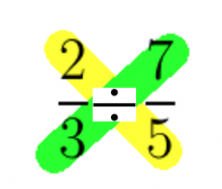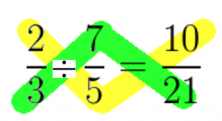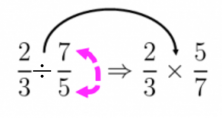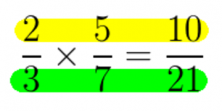Smartick is an online platform for children to master math in only 15 minutes a day

Jul14

# Learn How to Divide Fractions with Two Different Methods

In this post, we are going to learn how to divide fractions. In order to do so, I’m going to teach you two simpler ways that will help you divide fractions.

### Method 1 to Divide Fractions: Cross Multiplication

This method consists of multiplying the first fraction’s numerator by the second fraction’s denominator. Then we place that number in the numerator of the final answer. To get the final answer’s denominator, we have to switch gears and multiply the first fraction’s denominator by the second fraction’s numerator.

You’ll see why this method is called “cross multiplication” by looking at this diagram here:In yellow: The first fraction’s numerator is multiplied by the second fraction’s denominator. The resulting number is written in the numerator of the final answer.

In green: The first fraction’s denominator is multiplied by the second fraction’s numerator. The resulting number is written in the denominator of the final answer.### Method 2 to Divide Fractions: Inverse Multiplication

This method requires using the inverse of the SECOND FRACTION, or in other words, switch the numerator and the denominator around. After finding the inverse of the fraction, the two fractions can be multiplied.

Remember that in order to multiply fractions, they need to be lined up: Numerator by numerator and denominator by denominator.Following the example we see above, we have to inverse the second fraction, which means we switch the places of the 7 and the 5. Now, we can switch from division to multiplication.

To multiply the two fractions, we need to multiply in lines:  numerator by numerator and denominator by denominator.As you can see, we’ve gotten the same answers by using two different methods. Which one are you going to use?